{{ message }}
/ d3-ease Public

Easing functions for smooth animation.

# d3/d3-ease

Switch branches/tags
Could not load branches
Nothing to show

## Latest commit

`a091968`

## Files

Failed to load latest commit information.
Type
Name
Commit time
Jun 4, 2021
Jul 22, 2020
Aug 23, 2020
Jun 4, 2021
Jun 4, 2021
Aug 24, 2018
Jun 4, 2021
Jun 4, 2021
Jun 5, 2021
Jun 4, 2021

# d3-ease

Easing is a method of distorting time to control apparent motion in animation. It is most commonly used for slow-in, slow-out. By easing time, animated transitions are smoother and exhibit more plausible motion.

The easing types in this module implement the ease method, which takes a normalized time t and returns the corresponding “eased” time . Both the normalized time and the eased time are typically in the range [0,1], where 0 represents the start of the animation and 1 represents the end; some easing types, such as elastic, may return eased times slightly outside this range. A good easing type should return 0 if t = 0 and 1 if t = 1. See the easing explorer for a visual demonstration.

These easing types are largely based on work by Robert Penner.

## Installing

If you use npm, `npm install d3-ease`. You can also download the latest release on GitHub. For vanilla HTML in modern browsers, import d3-ease from Skypack:

```<script type="module">

import {easeCubic} from "https://cdn.skypack.dev/d3-ease@3";

const e = easeCubic(0.25);

</script>```

For legacy environments, you can load d3-ease’s UMD bundle from an npm-based CDN such as jsDelivr; a `d3` global is exported:

```<script src="https://cdn.jsdelivr.net/npm/d3-ease@3"></script>
<script>

const e = d3.easeCubic(0.25);

</script>```

Try d3-ease in your browser.

## API Reference

# ease(t)

Given the specified normalized time t, typically in the range [0,1], returns the “eased” time , also typically in [0,1]. 0 represents the start of the animation and 1 represents the end. A good implementation returns 0 if t = 0 and 1 if t = 1. See the easing explorer for a visual demonstration. For example, to apply cubic easing:

`const te = d3.easeCubic(t);`

Similarly, to apply custom elastic easing:

```// Before the animation starts, create your easing function.
const customElastic = d3.easeElastic.period(0.4);

// During the animation, apply the easing function.
const te = customElastic(t);```

# d3.easeLinear(t) <>

Linear easing; the identity function; linear(t) returns t.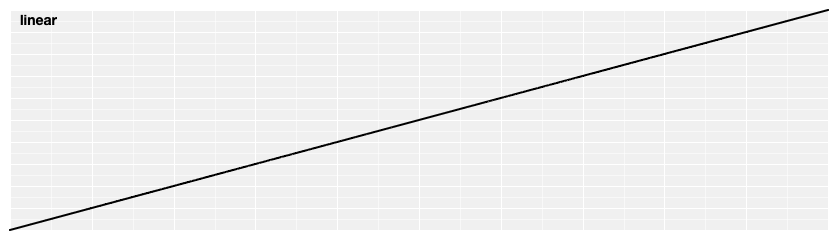# d3.easePolyIn(t) <>

Polynomial easing; raises t to the specified exponent. If the exponent is not specified, it defaults to 3, equivalent to cubicIn.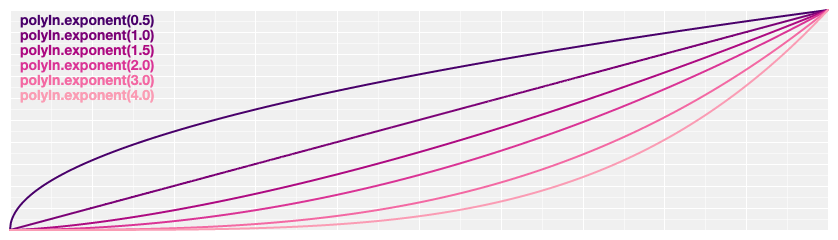# d3.easePolyOut(t) <>

Reverse polynomial easing; equivalent to 1 - polyIn(1 - t). If the exponent is not specified, it defaults to 3, equivalent to cubicOut.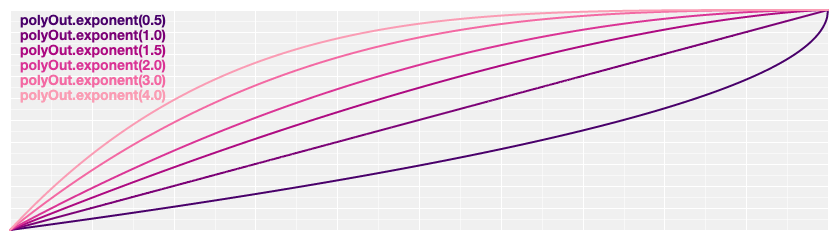# d3.easePoly(t) <>
# d3.easePolyInOut(t) <>

Symmetric polynomial easing; scales polyIn for t in [0, 0.5] and polyOut for t in [0.5, 1]. If the exponent is not specified, it defaults to 3, equivalent to cubic.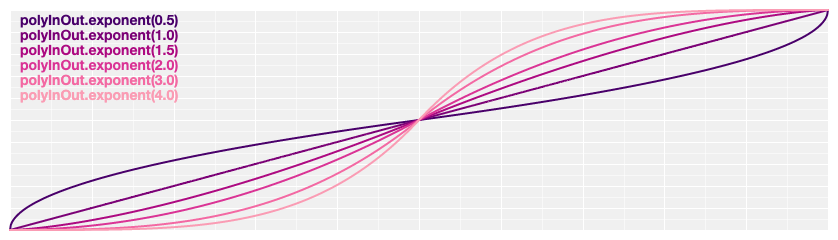# poly.exponent(e) <>

Returns a new polynomial easing with the specified exponent e. For example, to create equivalents of linear, quad, and cubic:

```const linear = d3.easePoly.exponent(1);
const quad = d3.easePoly.exponent(2);
const cubic = d3.easePoly.exponent(3);```

Quadratic easing; equivalent to polyIn.exponent(2).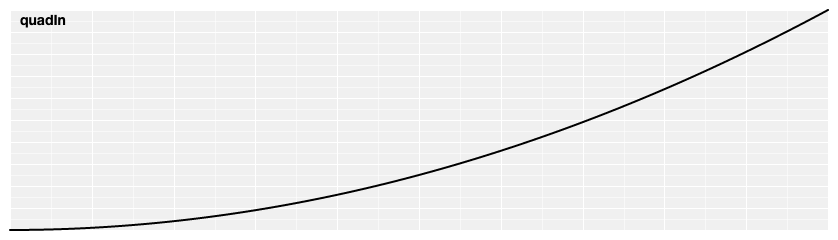Reverse quadratic easing; equivalent to 1 - quadIn(1 - t). Also equivalent to polyOut.exponent(2).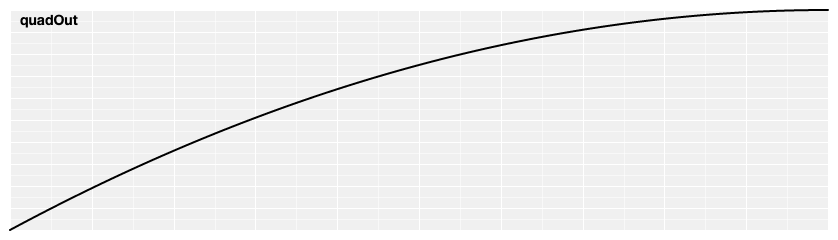Symmetric quadratic easing; scales quadIn for t in [0, 0.5] and quadOut for t in [0.5, 1]. Also equivalent to poly.exponent(2).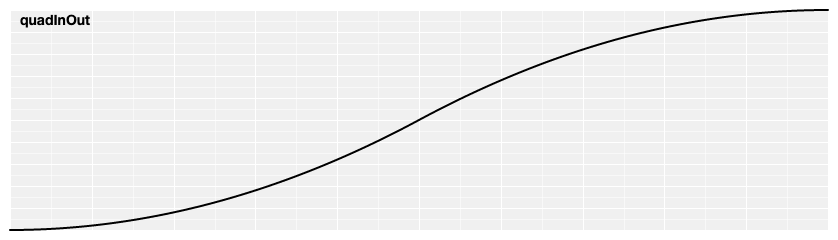# d3.easeCubicIn(t) <>

Cubic easing; equivalent to polyIn.exponent(3).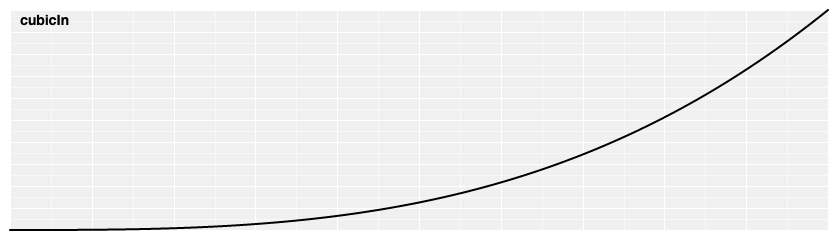# d3.easeCubicOut(t) <>

Reverse cubic easing; equivalent to 1 - cubicIn(1 - t). Also equivalent to polyOut.exponent(3).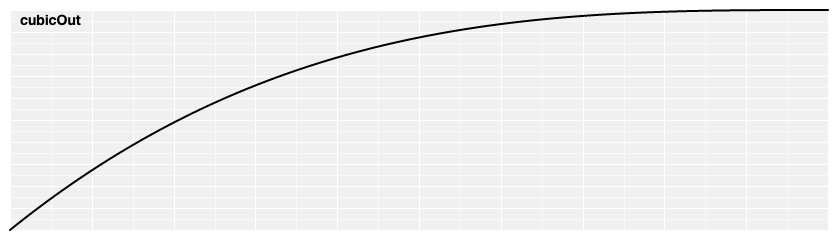# d3.easeCubic(t) <>
# d3.easeCubicInOut(t) <>

Symmetric cubic easing; scales cubicIn for t in [0, 0.5] and cubicOut for t in [0.5, 1]. Also equivalent to poly.exponent(3).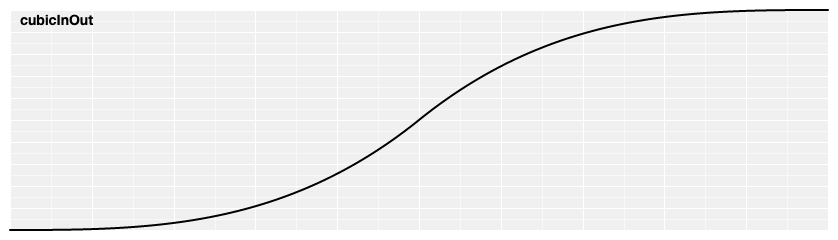# d3.easeSinIn(t) <>

Sinusoidal easing; returns sin(t).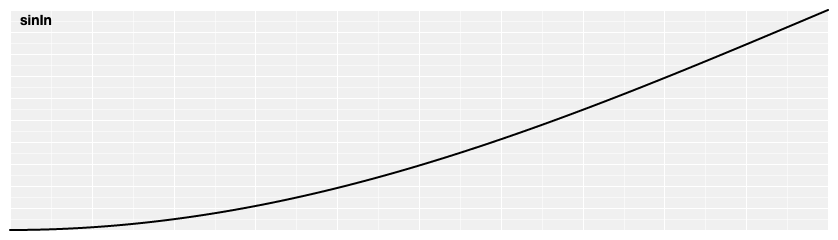# d3.easeSinOut(t) <>

Reverse sinusoidal easing; equivalent to 1 - sinIn(1 - t).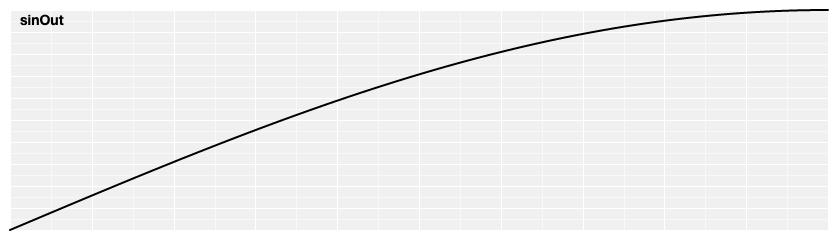# d3.easeSin(t) <>
# d3.easeSinInOut(t) <>

Symmetric sinusoidal easing; scales sinIn for t in [0, 0.5] and sinOut for t in [0.5, 1].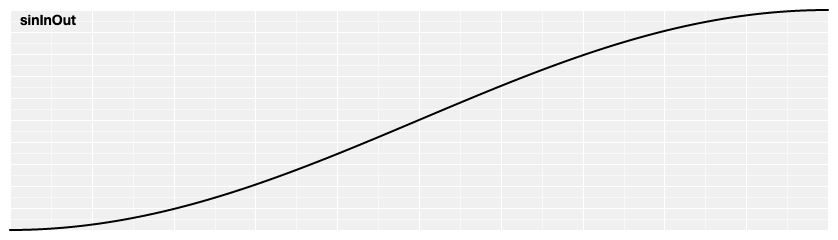# d3.easeExpIn(t) <>

Exponential easing; raises 2 to the exponent 10 * (t - 1).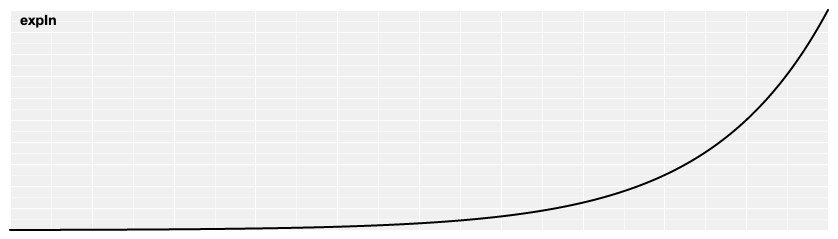# d3.easeExpOut(t) <>

Reverse exponential easing; equivalent to 1 - expIn(1 - t).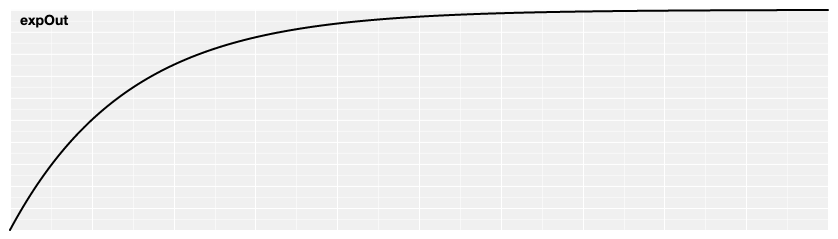# d3.easeExp(t) <>
# d3.easeExpInOut(t) <>

Symmetric exponential easing; scales expIn for t in [0, 0.5] and expOut for t in [0.5, 1].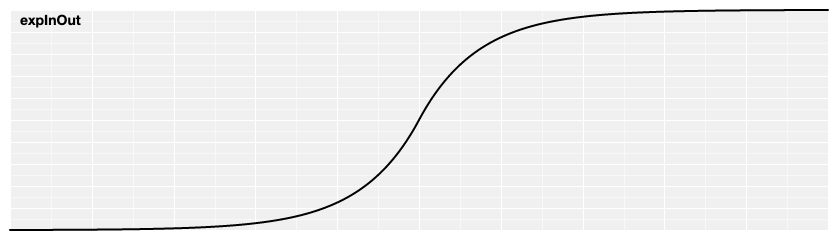# d3.easeCircleIn(t) <>

Circular easing.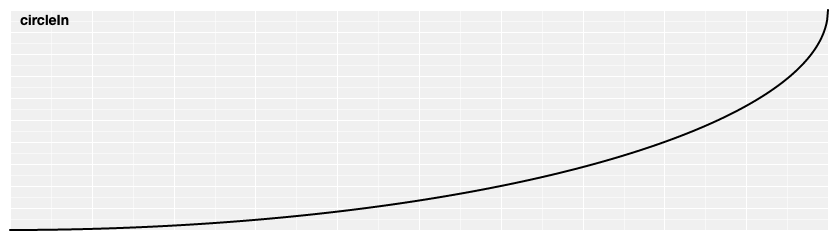# d3.easeCircleOut(t) <>

Reverse circular easing; equivalent to 1 - circleIn(1 - t).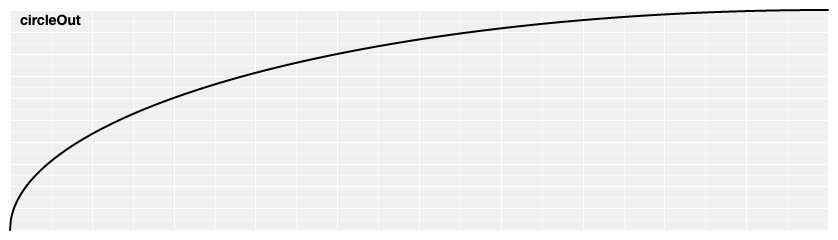# d3.easeCircle(t) <>
# d3.easeCircleInOut(t) <>

Symmetric circular easing; scales circleIn for t in [0, 0.5] and circleOut for t in [0.5, 1].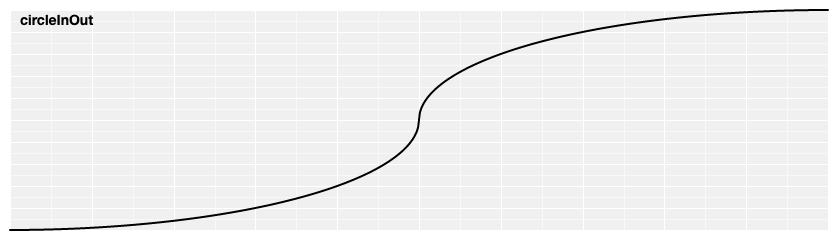# d3.easeElasticIn(t) <>

Elastic easing, like a rubber band. The amplitude and period of the oscillation are configurable; if not specified, they default to 1 and 0.3, respectively.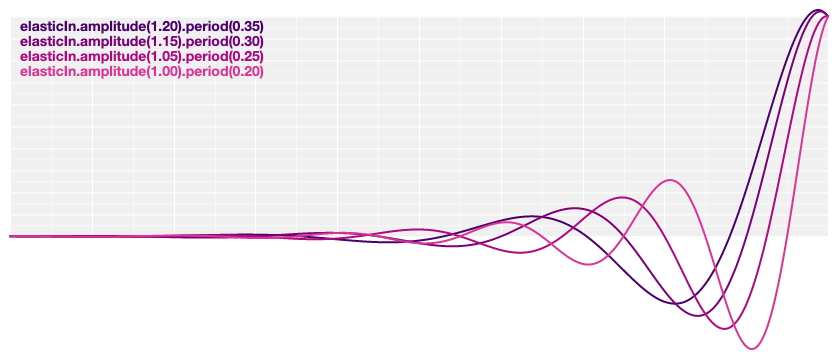# d3.easeElastic(t) <>
# d3.easeElasticOut(t) <>

Reverse elastic easing; equivalent to 1 - elasticIn(1 - t).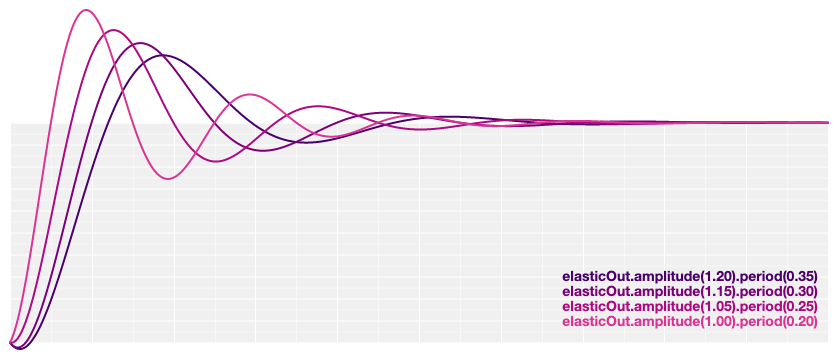# d3.easeElasticInOut(t) <>

Symmetric elastic easing; scales elasticIn for t in [0, 0.5] and elasticOut for t in [0.5, 1].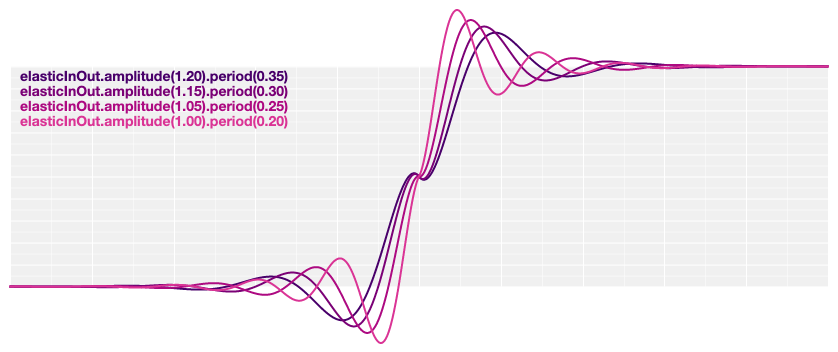# elastic.amplitude(a) <>

Returns a new elastic easing with the specified amplitude a.

# elastic.period(p) <>

Returns a new elastic easing with the specified period p.

# d3.easeBackIn(t) <>

Anticipatory easing, like a dancer bending his knees before jumping off the floor. The degree of overshoot is configurable; if not specified, it defaults to 1.70158.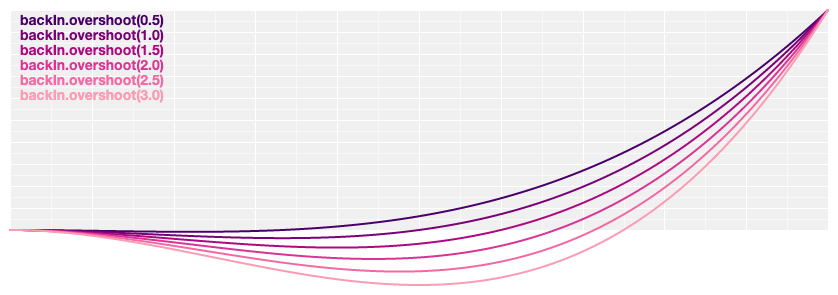# d3.easeBackOut(t) <>

Reverse anticipatory easing; equivalent to 1 - backIn(1 - t).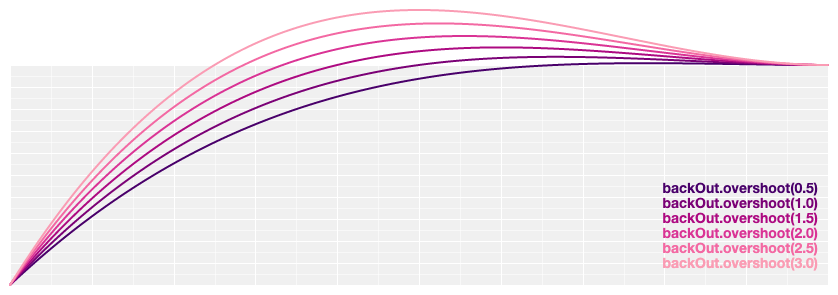# d3.easeBack(t) <>
# d3.easeBackInOut(t) <>

Symmetric anticipatory easing; scales backIn for t in [0, 0.5] and backOut for t in [0.5, 1].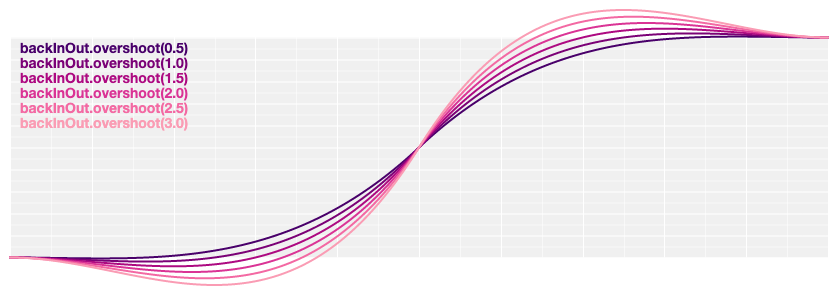# back.overshoot(s) <>

Returns a new back easing with the specified overshoot s.

# d3.easeBounceIn(t) <>

Bounce easing, like a rubber ball.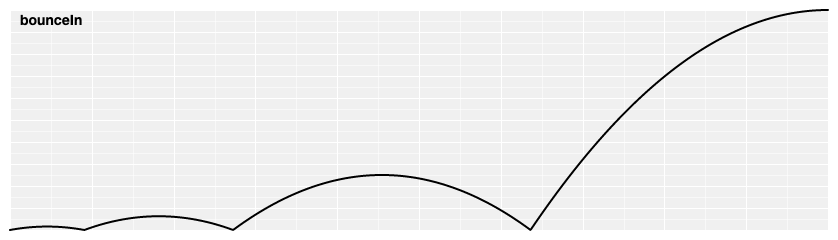# d3.easeBounce(t) <>
# d3.easeBounceOut(t) <>

Reverse bounce easing; equivalent to 1 - bounceIn(1 - t).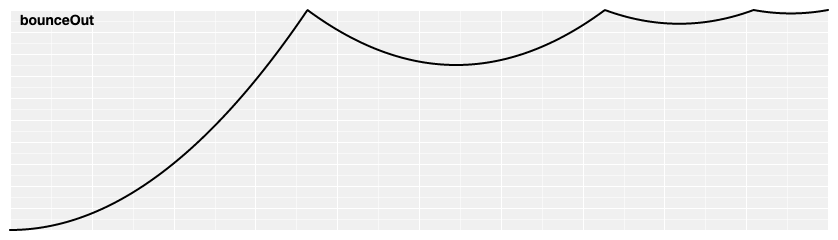# d3.easeBounceInOut(t) <>

Symmetric bounce easing; scales bounceIn for t in [0, 0.5] and bounceOut for t in [0.5, 1].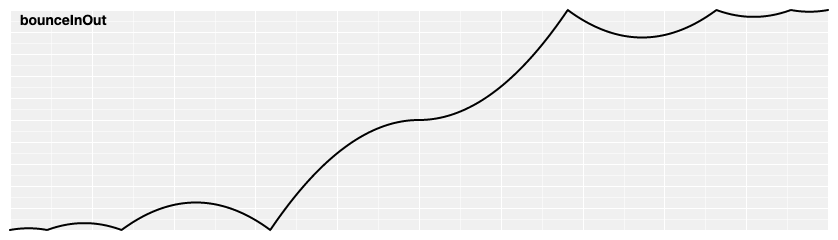Easing functions for smooth animation.

v3.0.1 Latest
Jun 5, 2021

## Packages 0

No packages published